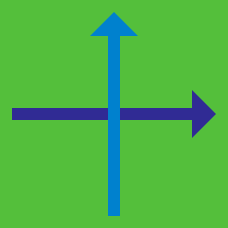Geometry

# Distance between point and line

If point $(p,0)$ is equidistant from the two lines $9x-y+1=0, x-9y-5=0$ and $p>0$, what is $p?$

Consider point $A=(a,2)$ with $a > 0$ such that over all points $P$ on the line $\frac{x}{4}+\frac{y}{3}=1,$ the minimum value of $\overline{AP}$ is $2.$ What is the value of $3a?$

Given a point $P$ on the positive $x$-axis such that the distance between $P$ and the line $4x+3y+6=0$ is $10,$ what are the coordinates of $P ?$

If real numbers $\ a\$ and $\ b\$ satisfy $a^2 + b^2 = 49$, what is the distance between the lines $ax + by = 1\;\;\; \textrm{and} \;\;\; ax + by = 225 ?$

If point $(p,0)$ is equidistant from the two lines $12x-y+1=0, x-12y-4=0$ and $p>0$, what is $p?$

×